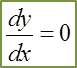# 9.7 Second-Order Differentiation, Turning Points, Maximum and Minimum Points

(A) Second-Order Differentiation

1. When a function y = x3 + x2 – 3x + 6 is differentiated with respect to x, the derivative  $\frac{dy}{dx}=3{x}^{2}+2x-3$

2. The second function   $\frac{dy}{dx}$ can be differentiated again with respect to x. This is called the second derivative of y with respect to and can be written as $\frac{{d}^{2}y}{d{x}^{2}}$ .

3. Take note that   $\frac{{d}^{2}y}{d{x}^{2}}\ne {\left(\frac{dy}{dx}\right)}^{2}$ .

For example,
If y = 4x3 – 7x2 + 5x – 1,

The first derivative   $\frac{dy}{dx}=12{x}^{2}-14x+5$

The second derivative    $\frac{{d}^{2}y}{d{x}^{2}}=24x-14$

(B) Turning Points, Maximum and Minimum Points(a) At Turning Points A and B,(b) At Maximum Point A(c) At Minimum Point B,Example 1 (Maximum Value of Quadratic Function)
Given that y = 3x (4 – x), calculate
(a) the value of x when y is a maximum,
(b) the maximum value of y.

Solution:
(a)

(b)

Example 2 (Determine the Turning Points and Second Derivative Test)
Find the coordinates of the turning points on the curve y = 2x3 + 3x2 – 12x + 7 and determine the nature of these turning points.

Solution:

6x2 + 6x – 12 = 0
x2 + x – 2 = 0
(x – 1) (x + 2) = 0
x = 1 or x = –2

When x = 1
y = 2(1)3 + 3(1)2 – 12(11) + 7
y = 0
(1, 0) is a turning point.

When x = –2
y = 2(–2)3 + 3(–2)2 – 12(–2) + 7
y = 27
(–2, 27) is a turning point.

Hence, the turning point (1, 0) is a minimum point.

Hence, the turning point (–2, 27) is a maximum point.